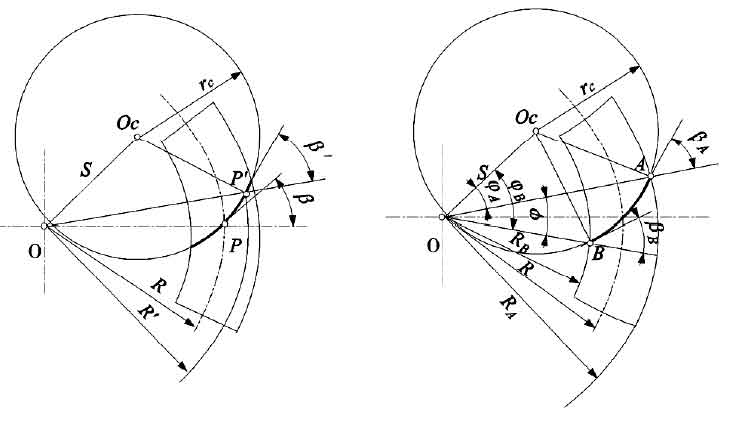# Spiral angle of spiral tooth non bevel gear

The inclination of spiral bevel gear teeth is measured by the helix angle β I. The longitudinal tooth profile of spiral bevel gear is the arc intersected by pitch plane and tooth surface, which is called pitch line, and the pitch line of plane gear is called tooth line. The angle between the tangent line of any point on the pitch line and the generatrix of the pitch cone is called the helix angle β I of the point.(a) helix angle of any point on gear pitch line (b) helix angle of small end and large end of gear

Generally, the spiral angle at the midpoint of pitch line is defined as the nominal helix angle β of spiral bevel gear, as shown in Fig. a. When the spiral bevel gear pair is meshed correctly, the big and small gears should have the same spiral angle besides the same pressure angle on the pitch line.

According to Δ oocp in Figure A and cosine theorem, it can be seen that:

Similarly, in Δ oocp ‘,

By subtracting the two formulas, the formula for calculating the helix angle at any point on the pitch line is as follows:

Where RC is the radius of the imaginary flat top gear.

The helix angles β A and β B of the large end and the small end can be obtained by the formula, as shown in Fig. B.